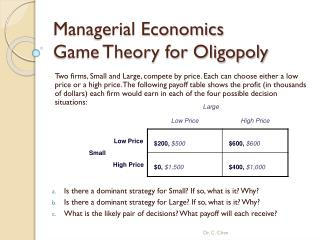# Managerial Economics Game Theory for Oligopoly - PowerPoint PPT PresentationDownload PresentationManagerial Economics Game Theory for Oligopoly

Managerial Economics Game Theory for OligopolyDownload Presentation## Managerial Economics Game Theory for Oligopoly

- - - - - - - - - - - - - - - - - - - - - - - - - - - E N D - - - - - - - - - - - - - - - - - - - - - - - - - - -
##### Presentation Transcript

1. Managerial Economics Game Theory for Oligopoly Two firms, Small and Large, compete by price. Each can choose either a low price or a high price. The following payoff table shows the profit (in thousands of dollars) each firm would earn in each of the four possible decision situations: • Is there a dominant strategy for Small? If so, what is it? Why? • Is there a dominant strategy for Large? If so, what is it? Why? • What is the likely pair of decisions? What payoff will each receive? Dr. C. Chen

2. Managerial Economics Game Theory for Oligopoly • Is there a dominant strategy for Small? If so, what is it? Why? • Play the game as Small. Given the rival‘s (Large) strategy of “Low Price”, Small will choose “Low Price” because the payoff \$200 is greater than \$0. Dr. C. Chen

3. Managerial Economics Game Theory for Oligopoly • Is there a dominant strategy for Small? If so, what is it? Why? • Again, given the rival‘s (Large) strategy of “High Price” , Small will choose “Low Price” because the payoff \$600 is greater than \$400. So, no matter what the rival’s strategy is, “Low Price” is the dominant strategy for Small. Dr. C. Chen

4. Managerial Economics Game Theory for Oligopoly b. Is there a dominant strategy for Large? If so, what is it? Why? Play the game as Large. Given the rival‘s (Small) strategy of “Low Price”, Large will choose “High Price” because the payoff \$600 is greater than \$500. Dr. C. Chen

5. Managerial Economics Game Theory for Oligopoly b. Is there a dominant strategy for Large? If so, what is it? Why? Then consider the rival‘s (Small) strategy of “High Price”. Large will choose “Low Price” to react because the payoff \$1,500 is greater than \$1,000. Therefore, there is no dominant strategy for Large. Dr. C. Chen

6. Managerial Economics Game Theory for Oligopoly c. What is the likely pair of decisions? What payoff will each receive? Small will always choose “Low Price” as the dominant strategy; therefore, Large will react by choosing “High Price”. The Nash equilibrium in this game will be (Small-Low Price, Large-High Price) associated with payoff \$600 for each. Dr. C. Chen